﻿ Electron correlation energy by configuration interaction (CI)

# Why "useless" quantum chemistry gives exact energy ?

## What are Pauli exclusion principle, and wavefunction ? → Shut up !

(Fig.1) ↓ Is this useful ?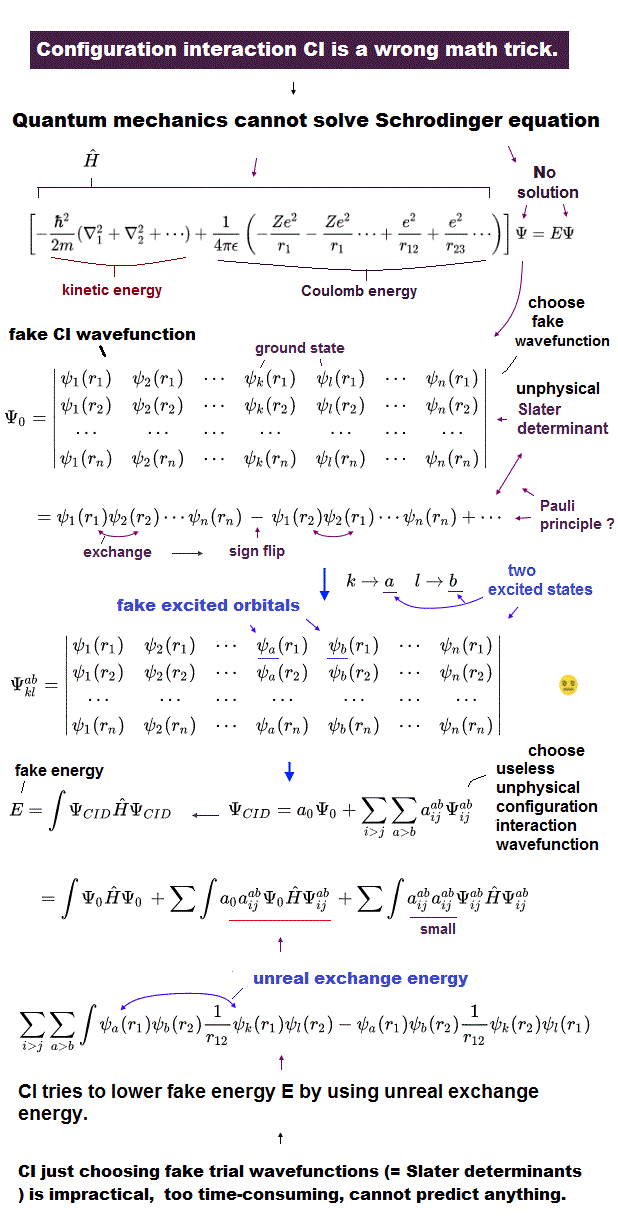As shown on this page, the present quantum chemistry NEVER tries to ask true mechanisms of Pauli exclusion principle and wavefunctions.
So, all we can rely on is Schrodinger equation and very complicated mathematical determinants.

In determinants like Fig.1, when two orbitals (= matrix lines ) are the same, this determinant becomes zero, which describes Pauli exclusion principle, they insist.

## Quantum chemistry is useless in actual molecules.

(Fig.1') One example -- doubly excited determinants.Even in small multi-electron atoms, we cannot solve Schrodinger equation exactly.
Dealing with large molecules such as protein interaction by Schrodinger equation is much more impossible.

But they just give up pursuing true machanism of wavefunction.
As shown on this page and this page, if we leave Pauli exclusion principle as it is (= only anticommutator of creation and annihilation operators ), imaginary concepts of spin, photon, phonons and virtual particles need to be created.

## Why quantum chemistry can give exact values ? → Shut up !

(Fig.2) Why quantum chemistry can give almost exact values ?Using complicated wavefunction of Fig.1 and variation methods, the quantum chemistry can give almost exact values in various atoms and molecules.
The important point is that most textbooks NEVER say about true reasons why the energies gotten by Schrodinger equation become close to experimental values.

They just say all we do is to blindly believe Schrodinger equation, and don't ask what the wavefunction really is.
But science should NOT be a religion.

In fact, the answer to these questions is these quantum mechanical methods completely rely on Coulomb energy and an integer times de Broglie wavelength through hydrogen-like wavefunctions ( 1s, 2s, 2p, ... ).
Using these hydrogen's wavefunctions ( with various energy levels ) and variation methods, we can get almost exact values in various atoms and molecules. This is the truth.

## An integer times de Broglie wavelength + variation are key players in quantum chemistry.

(Fig.3) de Broglie wavelength + variation methods = heart of quantum chemistry.Hartree-Fock method and configuration interaction in quantum chemistry use variational functions based on hydrogen atomic orbitals such as 1s, 2s, 2p, 3s, 3p ...
And each coefficient and charge Z are usually treated as variational parameters.
They find the lowest energies by varying those variational parameters.

The important point is that the concept of an integer multiple of de Broglie wavelength is indispensable, and used in these variational methods secretely, though most textbooks don't try to reveal this important fact.
As I explain later, hydrogen-like variational functions always keep their specific de Broglie wavelegnth while finding the proper charge Z.

## True mechanism of variation methods in quantum chemistry.

(Eq.1) Schrodinger wavefunctions.For example, we use 1s and 2p hydrogen like wavefunctions of Eq.1 to explain the mechanism of variation methods.
This Schrodinger's hydrogen like atom (= nuclear charge Z ) gives energy levels of

(Eq.2)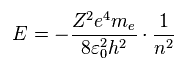All these energy levels are completely equal to those of Bohr model.
In Bohr model, the principal quantum number n=1 means this orbit is 1 × de Broglie wavelength.
Considering the same energies in them, Schrodinger's wavefunctions also obey an integer times de Broglie wavelength ?

In fact, as shown in this section, Schrodinger's wavefunctions always adopt the same concept of de Broglie wavelength as Bohr-Sommerfeld model !
Though most textbooks NEVER say this very important and true fact.

## Virial theorem

(Eq.3) Virial theorem.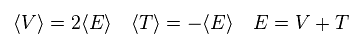As shown on this page, Schrodinger's hydrogens always satisfy Virial theorem like Bohr model.
In Eq.3, the average potential energy V is twice the total energy E.
The total energy E is the sum of potential energy V and the kinetic energy T.

From Eq.2 and Eq.3, the potential energy V is twice the energy E,
(Eq.4)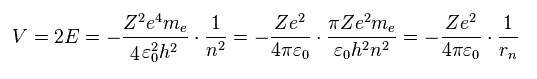where
(Eq.5)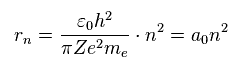rn is the average orbital radius both in Schrodinger's hydrogen and Bohr model.
"n" of Eq.5 is the principal quantum number, which means energy levels.
So, when n = 1 and n = 2, each average orbital radius becomes

## Average orbital radius (= distribution ).

(Fig.4) Orbital radius in each energy level.When nuclear charge Z = 1 and n = 1, the orbital radius a0 is called "Bohr radius", which is important value both in Schrodinger's hydrogen and Bohr model.

## Charge Z1 = variational parameter.   ← important.

(Eq.6) Variation functions 1s, 2p.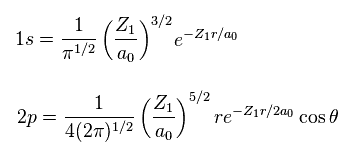In quantum chemistry, hydrogen-like wavefucntions including variational parameters Z1 are used.
These are called Slater-type orbitals, and variational change Z1 is called orbital exponent.

Eq.6 is 1s and 2p Slater-type orbitals.
Using Eq.6, we change parameter Z in wavefunction into Z1,
(Eq.7)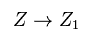Here we change only variational parameter Z ( → Z1 ) included in electron's wavefunction.
And the nuclear charge Z is not changed

## Distirubution (= radius ) changes by variation parameter.

(Fig.5) Changing parameter Z1 in wavefunction.Considering the situation of Fig.5, and substituting Z1 into Eq.4 and Eq.5, the orbital radius rn and potential V become
(Eq.8)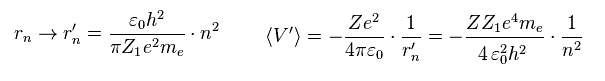And when Z ( → Z1 ) included in wavefunctions changes, average kinetic energy (= T ) becomes
( using Virial theorum T = -E )

## Kinetic energy changes by variation parameter.

(Eq.9) Average kinetic energy T.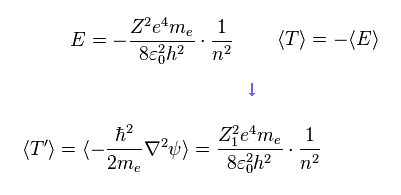In Eq.7, Eq.8, and Eq.9, only charge parameter Z included in the wavefunction itself is changed into Z1.
And the nuclear charge Z doesn't change. ( See Fig.6 )
Because charge Z1 in the wavefunction is used as variation parameter in quantum chemistry.

As a result, all Z in the kinetic energy T are changed into Z1, because the information of kinetic energy T is included inside wavefunction ( Eq.9 ).
And only one Z in the potential energy V is changed in to Z1, because one of Z in V originates from nuclear charge Z. ( See Eq.8. )

## Variation parameter changes, → radius and kinetic energy change.

(Fig.6) Changing variation parameter into Z1 inside wavefunction.New total energy E' is the sum of potential energy V' (= Eq.8 ) and kinetic energy T' (= Eq.9 ),
(Eq.10)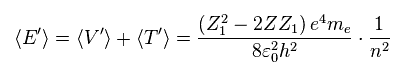Varying parameter Z1 in Eq.10, we find the total energy E becomes the lowest, when this parameter Z1 is just equal to nuclear charge Z, as follows,

## When variation parameter = true charge, total energy becomes the lowest.

(Eq.11) When Z1 = Z → total energy E becomes the lowest.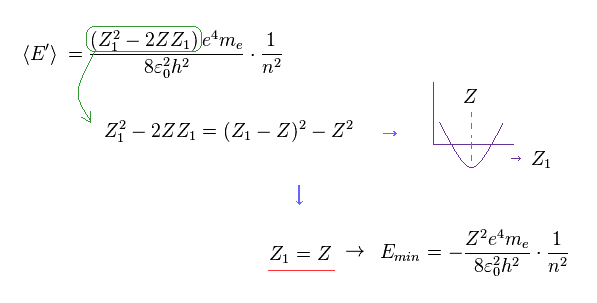This is the true mechanism in variational principle used in all ab-initio quantum mechanical methods such as Hartree-Fock and configuration interaction (= CI ).
The result of Eq.11 indicates that variational parameter Z1 included in the wavefunction needs to be the closest to the true charge Z which the electron feels.
And the important point is that this mechanism always applies in wavefunctions of all energy levels "n". ( See Eq.11. )

## Variation of 1s wavefunction = de Broglie wave is always "1".

(Fig.7) When variational parameter Z1 = Z, the energy E is the lowest and stable.Fig.7 is the summary of the mechanism used in variational methods.
For example, if you use 1s hydrogen like wavefunction, this wavefunction is always 1 × de Broglie wavelength.
And when the variational charge parameter Z1 is just equal to nuclear charge Z, which strength the electron feels, the total energy E becomes the lowest and stable.

When this Z1 inside wevefunction is bigger than Z ( Fig.7 left ), the kinetic energy T based on the wavefunction becomes too big. This increased kinetic energy T overcomes decreased potential energy V, so the total energy E is higher.
When Z1 is smaller than Z, the atomic radius becomes larger, which leads to higher potential energy V.
As a result, when Z1 = Z, the total energy E is the lowest.

## Variation using 2p wavefunction = de Broglie wave is always "2".

(Fig.8) When Z1 = Z → the energy E is the lowest also in n = 2 wavefunction.Een if you use 2p wavefunction ( n = 2 ), the variational mechanism of Fig.7 applies.
As shown in Eq.11, irrelevant to the principal quantum number "n", when Z1 inside wavefunction is just equal to nuclear charge Z, the total energy E is the lowest.

The main difference between 1s and 2p wavefunctions is 2p fucntion expresses 2 × de Broglie wavelength.
So if you use 2p wavefunction as trial function, the atomic radius tends to be bigger than 1s wavefunction.
( When you try to make atomic radius smaller by increasing Z1, the total energy E becomes higher and unstable. )

## Quantum mechanics dislikes "real" de Broglie waves, though it depends on them.   Why ?

(Fig.9) Angular momemtum L = 0 in S wavefunction → destructive interference of de Broglie wave !Of course, Schrodinger equations completely depend on de Broglie theory ( p = h/λ ) in calculating various energies.
In spite of this fact, quantum mechanics hates to admit real de Broglie wave. Why ?
And as shown in this section and this page, we can prove that Schrodinger's hydrogen also satisfies an integer number of de Broglie wavelength.

In Bohr-Sommerfeld model, the orbit becomes elliptic or circular, and cannot be linear ( L = 0 ).
Because when the angular momentum L is zero like S function, de Broglie waves with opposite phases cancel each other out.
" An integer times " means the wave phases of these de Broglie waves fit each other at their ends, and don't cancel.
( And the electron always collides with nucleus, when L = 0. This is impossible. )

## "S" wavefunctions cancel out their de Broglie waves !?

But in Schrodinger's hydrogen including S wavefunctions, the angular momentum L can be zero !
In these cases, the opposite phases of electron's de Broglie waves cancel each other out (= destructive interference ), and the electron is expelled from this orbit, according to Davisson-Germer interference expeimenrt.

This is one of reasons why they NEVER admit real de Broglie waves in quantum mechsnics, and strange many-world superposition becomes dominant in top journals even now.

## How "stationary" electron wavefunctions can repel each other ?

### [ True machanism of quantum mechanical helium. ]

(Eq.12) Schrodinger equation for helium atom.They insist quantum mechanics can give almost exact energy values in helium atom.
Of course, we cannot solve three-body helium atom, so we have to rely on some approximations.
Eq.12 is Hamiltonian (= total energy ) of helium atom.

Helium (= He ) contains two electrons (= 1, 2 ), and Z = 2 positive nucleus.
The first two terms of Eq.12 are kinetic energies of two electrons.
And the remaining terms are potential energy among Z=2 nucleus and two electrons.
r12 means the distance between two electrons.

## Helium 1s,1s wavefunction ?

(Eq.13) 1s × 1s wavefunctions in He.They use 1s type variational functions ( see Eq.6 ) in two electrons.
"Z1" is variational charge parameter. "a0" is Bohr radius.

From wavefunction of Eq.13 and Hamiltonian of Eq.12, total energy W becomes
(Eq. 14)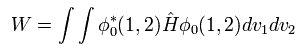By solving Eq.14, we obtain
(Eq. 15)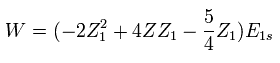In Eq.15, E1s is -13.606 eV.

## Charge Z1 is variation parameter.

We vary variational parameter Z1 to find the lowest energy W.
Differentiating W with respext to Z1,
(Eq.16)As shown in Eq.16, when variational parameter Z1 is 1.687, the total energy of this helium becomes the lowest.
(Eq.17)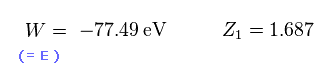The experimental value of ground state energy of helium is -79.005 eV.
Eq.17 is close to this experimental value, but a little different.
Because quantum mechanical stationary wavefunctions cannot treat with two electrons avoiding each other.
Qunatum mechanis hates particle's motion, and it just shows probability density.

For two negative electrons to avoid each other, we have to change stationary wavefunctions into dynamic waves.
But this dynamic wavefunctions clearly contradicts the basic concept of probability density in wavefunction.
As you feel, if we try to describe two electrons avoiding each other, we have to treat two electrons as real moving particles.

## Realistic helium model.

(Fig.10) Hydrogen and Helium atoms.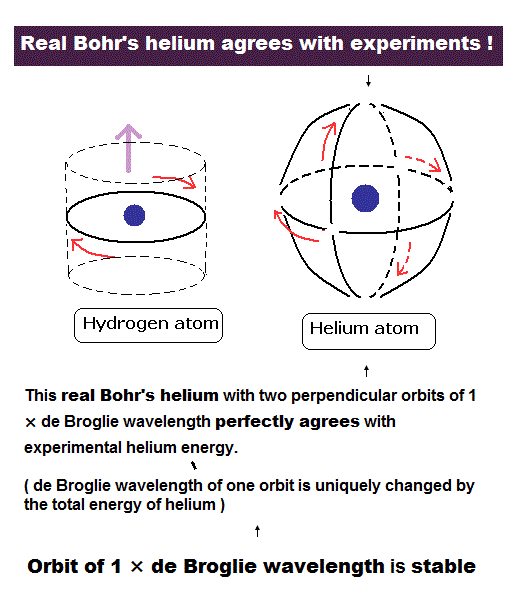As shown on top page, if we think two orbital planes of helium are perpendicular to each other, we can naturally obtain true ground state energies. ( -79.0037 eV, the slight difference of -0.0013 eV is relativistic effect, which is good. )
The reason why we have to make two orbits perpendicular to each other is to avoid detructive interference of two de Broglie waves.

So considering this desructive interference of de Broglie waves, Pauli exclusion principle (= third electron of Li cannot enter 1s orbit ) can be naturally explained by this new Bohr model.
Spin-Spin interaction is too small (= fine structrue level, 0.00005 eV ) to overcome Coulomb attractive force, because Pauli exclusion principle is very strong repulsive effects (= greater than 10 eV in Li ).

(Table 1) Calculated results of two electron atoms by new Bohr model.
Atoms r1 (MM) WN x 4 Circular orbit Result (eV) ExperimentError (eV)
He 3074.0 1.000000 -83.335-79.0037 -79.0051 0.001
Li+ 1944.5 1.000000 -205.78-198.984 -198.093-0.89
Be2+ 1422.0 1.000000 -382.66-373.470 -371.615-1.85
B3+ 1121.0 1.000000 -613.96-602.32 -599.60-2.72
C4+ 925.0 1.000000 -899.67-885.6 -882.1-3.50
N5+ 788.0 1.000000 -1239.8-1223.3 -1219.1-4.20
O6+ 685.3 1.000000 -1634.38-1615.44 -1610.70-4.74
F7+ 607.3 1.000000 -2083.3-2062.0 -2057.0-5.00
Ne8+ 544.5 1.000000 -2586.7-2563.0 -2558.0-5.00

Surprisingly, this new atomic structure of Bohr's helium is applicable to all other two and three electron atoms ( ions ).

## True mechanism in helium variational methods.

(Fig.11) Variation parameter Z1 needs to be equal to true charge.As I said in Eq.17, when variational parameter Z1 is 1.687, this ground state energy becomes the lowest (= -77.49 eV ).
This value of 1.687 is proper, because due to another electron's existence, the central charge which each electron feels becomes less than Z = 2.

If you compute total energy, by substituting Z1 = 2 instead of 1.687, you will find the energy becomes higher (= -74.83 eV ) than -77.49 eV. ( See also this page. )
This is the same pattern as Fig.7.
True central charge always becomes less than "2" due to the existence of another electron.
If you adopt wrong charge parameter inside wavefunction, this energy becomes higher due to the increased kinetic energy.

## How electron correlation can be described in helium atom ?

(Fig.12) Probability density of each electrons is changing depending on r12 ?As shown in Eq.17, the variational method cannot give correct ground state energy of helium.
Because Schrodinger's wavefunction cannot treat two electrons, which are moving around to avoid each other.

So in 1928-1930, Hylleraas used the special wavefunctions containing the interelectronic distance r12, as shown in Fig.12.
This wavefunction becomes larger, as this interelectronic distance r12 is longer.
So, as you notice, this Hylleraas wavefunction of Fig.12 is NOT stationary.
This Non-stationary wavefunction clearly contradicts the probability concept of wavefunction.

## Hylleraas wavefunction is dynamical !?

(Eq.18)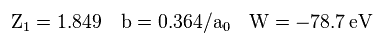The calculated result of Fig.12 becomes -78.7 eV, which is lower than -77.49 eV of Eq.17, but still higher than true experimental energy (= -79.005 eV ).
And depending on interelectronic distance r12, the probability density at each point changes, which is very strange.

(Eq.19)To get the ground state energy close to the experimental value, we have to use more than 1000 variational functions (= very unrealistic ! ) like Eq.19.
So these quantum mechanical methods have already reached their limits even in simple helium atom.
Furthermore, the latest computed value of -79.015 eV is different from the experimental value of -79.005 eV.
Because, wavefunction cannot consider correct nuclear motion (= reduced mass ) in helium atom.

## Electron correlation 2 --- Superposition ??

(Eq.20) Each electron = "superposition" of various wavefunctions ?Hylleraas fucntion containg interelectronic distance is too complicated to be applied to larger atoms.
So in larger atoms, they often use configuration interaction (= CI ) method.
In CI, atomic orbital is in the superposition state consisting of various wavefunctions ( with various principal quantum number ).

For example, in Eq.20, each electron's wavefunction consists of five different variational functions ( Clementi and Roetti ).
Surprisingly, these variational functions includes unrealistic charges (= 2.377, 4.396, 6.527 .. ).
Helium has Z=2 positive nuclear charge, so Z > 2 charges are impossible.
This is a fatal defect in unrealistic variational methods.

## Two different radii (= Z ) express two electrons avoid each other ?

(Eq.21) Combination of wavefunctions with different atomic radii in helium ( Z = 2 ).As a result, this helium wavefunction includes different combinations with different atomic radii.
For example, in Eq.21, atomic radius in Z1 = 1.417 is much larger than that in Z1 = 7.942.
( As I said. it is impossible that Z=2 helium includes charge Z1=7.942 from the realistic viewpoint. )

The calculated result of Eq.20 becomes -77.9 eV, which is a little lower than -77.49 eV in Eq.17 (= not superposision ).

(Fig.13) Two electrons avoid each other ?As a result, in the superposition of Eq.20, repulsive interaction between two electrons becomes weaker in some terms containing different-type wavefunctions.
This variational parameter of Z5 = 7.942 ( ← unrealistic ! ) is much larger than real helium nuclear charge Z=2, so the computed energy becomes higher only in each single wavfunction, as I said in Fig.7.

But due to the existence of terms consisting of different-sized orbitals, repulsive potential between two electrons becomes lower, which leads to the lower total energy.
This is the true mechanism in all of the present quantum chemistry ( ab-initio ).
As you feel, they NEVER admit each electron as clearly separated particle, these wavefunctions become extremely complicated and out of touch with reality. ( → Shut up and calculate ! )

## What causes Pauli exclusion principle in Lithium atom ?

(Eq.22) Lithium ( Z = 3 ) Hamiltonian.Next we treat lithium atom (= Li ), which has Z = +3e positive nucleus, two 1s electrons, and one 2s electron.
So the Hamiltonian (= total energy E ) becomes as shown in Eq.22.

(Eq.23) Slater type (= hydrogen ) orbitals.1s and 2s wavefunctions originate in hydrogen atom.
Z1 and Z2 are variational charge parameters, so this case in Li is also the same as Fig.7 and Fig.8.

(Eq.24) If all three electrons in Li are in 1s orbital ....The important point is that if we try to consider all three orbitals in Eq.24 are 1s wavefunctions, the calculated value of Li ground state energy becomes less than -214.3 eV, which is lower than the experimental value (= -203.5 eV )

So this result completely violates the variation principle, because the calculated value is already lower than the experimental value before we do variational methods.
As a result, the idea that all three Li electrons are in 1s state is completely wrong.

As you see the result of Eq.24, Li atom uses Pauli exclusion principle as strong repulsive force.
This repulsive force of Pauli exclusion principle is more than 10 eV, which is much bigger than usual spin-spin interaction (= fine structure level, 0.00005 eV ).
So Pauli exclusion principle can NEVER be caused by spin-spin interaction.

## Pauli exclusion principle = abstract determinant ?

(Eq.25) Pauli exclusion principle = determinant ??So they introduced mathematical determinant to describe Pauli exclusion principle.
In determinant, when two columns or lines are the same (= for example 1s = 2s ), this wavefunction becomes zero.
So 2s function of Eq.25 cannot be 1s, they insist.

But as you feel, this determinant just forbids 2s to be equal to 1s, "mathematically" ( NOT physically ).
As shown on this page, even in relativistic quantum field theory, they only shows very abstract anticommutator, NOT showing any more concrete thing.

This vague and unkown Pauli exclusion principle (= anticommutator ) is the main reason why the present quantum field theory ( including condensed matter and particle physics ) is completely useless.

(Eq.26)Using Eq.25 in Eq.22, the calculated value becomes -201.2 eV.
As shown on this page, our new Bohr model can give the ground state energy of -203.033 eV, which is closer to the experimental value of -203.5 eV.

As I said in Eq.25, spin-spin interaction is too weak to cause Pauli exclusion principle, overcoming Coulomb attractive force.
So there is only one thing left to explain this important repulsive force of Pauli exclusion.
As shown on this page, de Broglie wave interference is the main reason of this Pauli exclusion principle.

## Various molecules by quantum chemistry.

### [ Hydrogen molecule ion ( H2+ ) by quantum chemistry is unrealistic. ]

(Eq.27) Hamiltonian of hydrogen molecule ion (H2+)Hydrogen molecule ion (H2+) consists of two +e nuclei and one electron.
Eq.27 is the Hamiltonian of this H2+.
Using 1s hydrogen wavefunction, H2+ wavefunction becomes

(Eq.28) H2+ wavefunction ?Z1 is variation parameter, and considering symmetry, wavefunction ψ is supposed to be the sum of wavefunctions based on nuclei 1 and 2.
Using Eq.28 in Eq.27, we get

(Eq.29) Internuclear distance (= Re ), dissociation energy (= De ).When variational parameter is Z1 is 1.24, internuclear distance (= Re) between n1 and n2 is 1.06 Å.
And dissociation energy (= De ) is 2.25 eV.
Eq.29 result is a little different from the experimetal value of

(Eq.30) Experimental value of H2+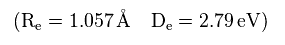As I said in Fig.7, 1s wavefunction always keeps 1 × de Broglie wavelength.
And when the variational parameter Z1 is just equal to the true charge which the electron feels, the calculated energy becomes the lowest.
( Z1 = 1.24 is a little bigger than 1, and less than 2. which is good. )

(Fig.7) When variational parameter Z1 = Z, the energy E is the lowest and stable.So H2+ ion by quantum chemistry also depends on an interger times de Broglie waves, which is the same mechanism as Bohr Sommerfeld model.
The result of Eq.29 is still different from the experimental value of Eq.30, so they combine 1s and 2s hydrogen wavefunctions like Eq.31. ( Dickinson. )

## Quantum variation of H2+ gives "unreal" charge parameter.

(Eq.31) H2+ ion wavefunction ?where
(Eq.32)Using Eq.31 and Eq.32 in Eq.27, the calculated result becomes

(Eq.33) H2+ consists of two protons → This charge Z2 is correct ?When the charge parameters are Eq.33, the energy becomes lowest ( dissociation energy = 2.73 eV ), which is closer to the experimental value.
But the important point is that charge parameter Z2 included in 2p variational function becomes unrealistic value (= +2.965 e ).

H2+ molcule ion has only two positive nuclei (= 2 × +e ), so this charge of +2.965 e is impossible, and shows quantum chemistry loses touch with reality.

Of course, as shown in Fig.8, 2p wavefunction always keeps 2 × de Brolgie wave, and gives the lowest energy, when Z2 is real charge ( < 2 ).
But in this case of H2+ ion, this 2p function needs to extend into another nucleus, and be as close to both nuclei as possible.
When Z2 is lower than real value of "2", this wavfunction is far away from another nucleus.
( But of course, Z2 > 2 is impossible from the realistic viewpoint. )

## Antisymmetric wavefunction is real ?

(Fig.14) H2+ wavefunction can be symmetric and antisymmetric ??If you see some textbooks, you will find H2+ ion wavefunction consists of symmetric (= Fig.14 upper ) and antisymmetric (= Fig.14 lower ) wavefunctions.
But from the realistic viewpoint, antisymmetric wavefunction is just mathematical tool, and unreal.

(Fig.15) Symmetric wavefunction.When H2+ wavefunction is symmetric, variation parameter Z1 is close to 2, when two nuclei are close to each other.

(Fig.16) Antisymmetric wavefunction is unreal.On the other hand, in case of antisymmetric wavefunction, this charge variation parameter Z1 becomes 0.4, when two nuclei stick to each other.
This is clearly unrealistic.
This antisymmetric wavefunction is often used in configuration interaction, because it can express virtual repulsive force among electrons.

So the present quantum chemistry is just mathematical thing.
In fact, when you ask if these wavefunctions are true or not, they only say "Shut up and calculate !", and don't try to explain the physical meaning of them.

## Repulsive interation between two electrons is weak point of quantum wavefunction.

(Eq.34) Hydrogen molecule (H2) HamiltonianHydrogen molecule consists of two +e nuclei and two electrons.
Valence bond ( VB = Heitler-London ) method can give more correct value than MO method.
So most textbooks often refer to this method.

In fact, this Heiter-London method disobeys Virial theorem.
The wavefunction of H2 is

(Eq.35) H2 wavefunction by Heitler-London methods.Using Eq.35 in Eq.34, we get
(Eq.36)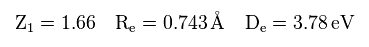Dissociation energy of Eq.36 is different from the experimental value of

(Eq.37) Experimental values of H2.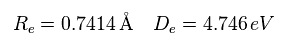Because H2 molecule includes repulsive interaction between two electrons.
As I said in helium section, these stationary wavefunctions need to be dynamic for two electrons to avoid each other.
This dynamic probability density clearly violates the original meaning of quantum wavefunction.

## More than 100 wavefunctions are needed for one H2 molecule !?

(Eq.38) Real hydrogen molecule ? = more than 100 terms.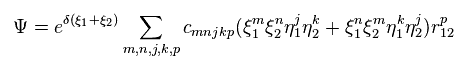To get the exact values, we have to use very complicated wavefunctions including variables such as r12.
" r12 " means the distance between two electrons, so the probability at which we find the electron 1 is changing depending on the position of electron 2.

As shown in this page, the idea that wavefunctions show probability density holds only for one-electron hydrogen atom.

## Configuration interaction relies on an integer times de Broglie waves.

### [ Unrealistic superposition = electron correlation ? ]

(Eq.39) Combining multiple excited wavefunctions ← useful as real sicence ?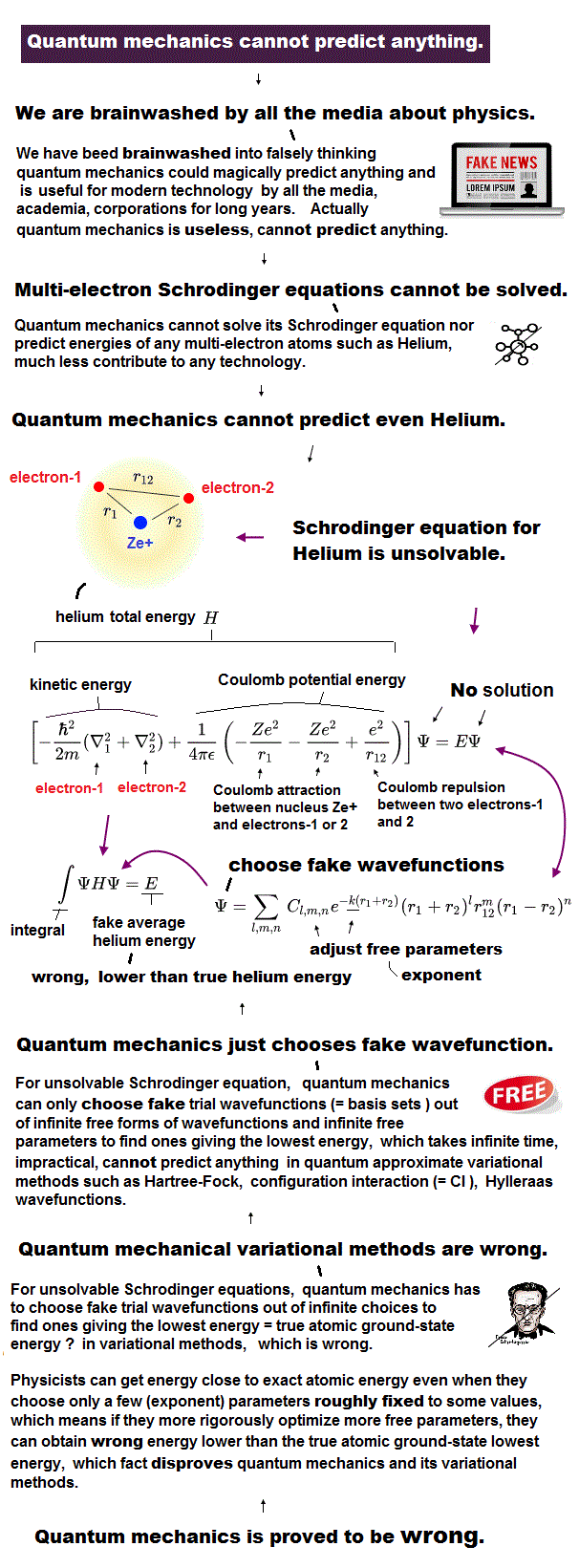To overcome the deficiencies of the Hartree-Fock wave function ( ex. electron correlation ), configuration interaction ( CI ) was introduced.
All ab-initio methods in quantum chemistry are based on this CI.
Each configulation function (= CSF ) is a linear combination of Slater determinants.

## ↓ Is this useful ?

(Eq.40) Doubly excited determinants.CI uses virtual excited orbitals such as singly excited (= Eq.39 ), doubly excited (= Eq.40 ) and triply excited.
As you see, these functions are too compilcated and disgusting.

(Eq.41) Electrons avoid each other ?Then, why CI can give the calculated results close to the experimental values ?
One reason is that they are completely based on the same mechanism of an integer times de Brolgie waves and variation through Slater type (= hydrogen atom ) orbitals, as I said in Fig.7 and Fig.8.

And when we combine various excited wavefunctions, two electrons with different orbitals can avoid each other.
By increasing various terms, CI can find proper wavefunctions, which can give the values close to experiment.
But as shown in Eq.21 (= He CI ) and Eq.33 (= H2+ CI ), the variational charge parameters (= Z2 ) of excited virtual orbitals tend to be unrealistic.
( In H2+ case, Z2 = 2.965 is greter than the sum of two +e nuclei. )

(Eq.42) CI wavefunctions.And as shown in Eq.39, Eq.40, Eq.42, this CI determinants are too complicated to be applied to larger molecules.
So we have to seek more realistic and simpler methods to express repulsive electrons.
To do so, first, we must ask what the wavefunctions and Pauli exclusion principle really are.

## Double zeta basis set. What is 6-31G ?

(Eq.43) STO and GTO functionsSlater-type orbitals (= STO ) originate in hydrongen atom, which can give more accurate energies than Gauss-type orbitals (= GTO ).
But GTO basis set can be computed more easily than STO.

(Eq.44)To use GTO basis set, we have to express STO function as a linear combinations of GTO functions.

(Eq.45)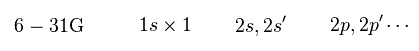6-31 G method uses 6 × GTO in inner shell, and 3 × GTO in outer shell.
And outer shells contain two different orbitals in each wavefunction.
The variation parameters and coefficients of these wavefunctions are determined in advance to express the energies close to the experimental value.

So 6-31G also depends on the same mechanism of an integer times de Broglie wavelength and variation as CI.

## Moller-Plesset ( MP ) perturbation can give wrong ground state energy.

(Eq.46)CI method takes extremely much time, so much simpler MP method was introduced.
For example, MP2 ( or MBPT2 ) uses second-order perturbation of energy, as shown in Eq.46.
Virtual energies (= a, b ) are higher than the original energies (= i, j ), so this second-order perturbation always negative.

As a result, MP2 always gives lower ground state energy than Hartree-Fock.
The inportant point is that MP2 can give wrong ground state energy, which is lower than the true energy.
( Of course, if the calculated ground state energy is lower than the actual energy, this theory is wrong. )

## Schrodinger equation is equal to Bohr-Sommerfeld model, again.

### [ Schrodinger wavefunctions also satisfy an integer times de Broglie wavelength. ]

(Fig.17) 1s hydrogen wavefunction = 1 × de Broglie wavelength.If you check hydrogen wavefunction based on Schrodinger equation in some websites or textbooks, you will find 1s radial wavefunction like Fig.17.
rR12 shows 1/2 de Broglie wavelength (= Fig.17 right ), so one orbit ( ∞ → 0 → ∞ ) includes 1 × de Broglie wavelength in the radial direction.

(Fig.18) 2s hydrogen wavefunction = 2 × de Broglie wavelength.In case of 2s wavefunction, it includes 1 × de Broglie wavelegnth (= one crest + one trough ) from 0 to ∞.
So one round orbit ( ∞ → 0 → ∞ ) contains 2 × de Broglie wavelegnth.

As shown on this page, hydrogen n=1 and n=2 orbits in Bohr model are 1 × and 2 × de Broglie wavelength, respectively.
So both Schrodinger and Bohr model hydrogens satisfy an integer times de Broglie waves,

(Fig.18') Schrodinger equation for hydrogen atom.The reason why χ = rR satisfies an integer times de Broglie wavelength is shown in Schrodinger equation of Fig.18'.
When we replace radial function R by rR, radial equation just expresses de Broglie momentum relation.

(Fig.19) Probability density in 6-s hydrogen wavefunction.Fig.19 shows the probability density (= a square of amplitude ) of 6s hydrogen wavefunction.
It is known that the number of nodes becomes ( n - l ) in probability functions.
( "n" is the principal quantum number, and "l" is the angular momentum. ).

So in 6s probability wavefunction ( n = 6, l = 0 ), the number of nodes is 6 - 0 = 6.
As a result, the wave amplitude (= rR6s ) consists of 3 × crest and 3 × trough, which means 3 × de Broglie wavelength from 0 to ∞.
This means one orbit ( ∞ → 0 → ∞ ) contains 6 × de Brolgie wavelength, which number is just equal to Bohr model.

## Sommerfeld quantization condition.

(Eq.45) Sommerfeld quantization condition (A) + de Broglie relation (B).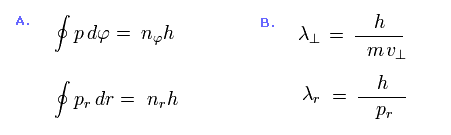Eq.45 shows Bohr-Sommerfeld quantization condition.
In Eq.45A, "p" denotes angular momentum, and "pr" denotes the radial momentum.
Eq.45B show tangental (= upper ) and radial (= lower ) de Broglie wavelengths. (= λ )

(Eq.46) Sommerfeld quantization = integer times de Broglie wavelength.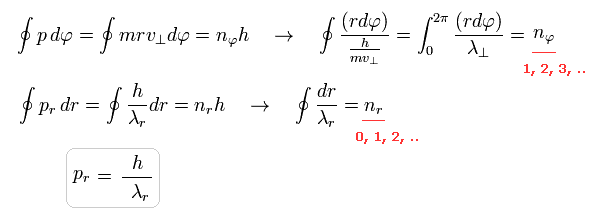Substituting de Broglie relation of Eq.45B into Sommerfeld conditions of Eq.45A, we find that Sommerfeld conditions mean an integer times de Broglie wavelength in both tangential and radial directions.

(Fig.20) 2p radial wavefunction = 1 × de Broglie wavelegnth.   Angular momentum = 1.As shown in Fig.20, 2p hydrogen wavefunction includes one crest, so one round orbit becomes 1 × de Broglie wavelegnth.
Though 2p is n=2 energy level, why 2p radial orbit contains only 1 × de Broglie wavelegnth ?
( Compare Fig.20 right with Fig.18 right )

Because 2p contains angular momentum ( l = 1 ), so one round tangential orbit also contains 1 × de Broglie wavelegnth. ( phase returns by φ = 0 → 2π )
As a result, the sum of radial and tangential waves becomes "2", which is consistent with n = 2 energy levels.

### [ Radial de Broglie waves. ]

(Eq.47) Sommerfeld quantization = integer times de Broglie wavelength.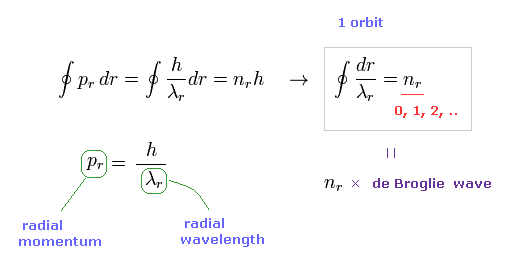Basically, angular momentum of Schrodinger equation is also quantized as an integer times ħ, which is the same as Bohr model.
So you can easily understant the tangential direction in Schrodinger's hydrogen also satisfies an integer times de Broglie wavelength.

The important point is that also in the radial direction ( of 1s, 2s, 2p .. ), an integer times de Broglie wavelength is satisfied in Schrodinger equation.
Radial de Broglie wavelength (= λr ) is expressed using radial momentum (= pr ), as shown in Eq.47.

(Eq.48) Sommerfeld model and 2p Schrodinger radial wavefunction.As shown in Eq.48, one round orbit means A (= aphelion ) → B (= perihelion ) → A (= aphelion ).
At points A and B of elliptical orbits, the radial momentum become zero ( pr = 0 ).
So according to de Broglie relation of Eq.47, radial wavelength becomes infinite at these points ( λr → ∞ ).

In 2p radial function, at both ends ( r = 0 and r = ∞ ), the wave phases stop, which means de Broglie wavelength becomes infinite.
( = the slopes of wavefunction become zero at both ends. )

(Eq.49) Bohr model and Schrodinger's hydrogen.Eq.49 upper is Bohr model equation, and Eq.49 lower is Schrodinger's Hamiltonian.
The momentum p is changed into space derivative in Schrodinger equation.
Both hydrogens use conpletely the same relation ( E = T + V ).

(Eq.50) One orbit of elliptical orbit.As shown in Eq.50, one round orbit means A (= aphelion ) → B (= pelihelion ) → A (= aphelion ).
And in hydrogen atom, orbital shape is symmetric, so one orbit can be expressed as 2 × ( A → B ).

In Eq.50, the radius at perihelion is r1, and that at aphelion is r2.
So radial coodinate r is between r1 and r2.
( Of course, in circular orbit, the radial motion is competely zero, and need NOT to be considered. )

(Eq.51) One orbit (= left ) and a half orbit (= right ).When one orbit contains 1 × de Broglie wavelength, a half orbit ( A → B ) contains 0.5 × de Broglie wavelength.

(Eq.52) Mometum p = first derivative ∇As I said in Eq.49, momentum p is replaced by a first derivative (= ∇ ) in Schrodinger equation.

(Eq.53) de Broglie relation.At perihelion and aphelion, the radial momentums become zero.
In Schrodinger hydrogen, zero momentum means wavelength λ is infinite.
So if you find the ends where de Broglie wavelength is infinite, they are perihelion and aphelion in Schrodinger's hydrogen.In 2p radial wavefunction, de Broglie wavelength becomes infinite at r=0 and r=∞.
Because at these points, the first derivative (= momentum ) becomes zero. ( slope is zero ).

(Eq.55)As shown in Eq.55, as wavelength λ is closer to infinity, the phase change becomes zero (= Eq.55 right ).

(Eq.56) A first derivative = momentum p.Eq.56 shows a first derivative of 2p wavefunctoin with respect to r, which means radial momentum pr.
As shonw in Eq.56 and Eq.57, this derivative becomes zero at both ends of r=0 and r=∞.

(Eq.57)Eq.57 means a half orbit ( from perihelion to aphelion ) in Schrodinger's hydrogen is from r=0 and r=∞.
As shown in Eq.54, this area contains 0.5 × de Broglie wavelength.
So one round orbit is 1 × de Brolgie wavelength in the radial direction.

### [ At the point of mometum p=0, kinetic energy (= p2 ) isn't always zero. ]

(Eq.58)The important point is that at the point of momentum p = 0, kinetic energy (= p2 ) doesn't become zero in Schrodinger equation.
This is very strange, because when p=0, p2 is NOT zero.

As shonw in Eq.58, the points where p2 (= second derivative ) is zero, are NOT equal to r =0 and r = ∞.

(Eq.59)Of course, Bohr Sommerfeld quantization conditions of Eq.47 uses first-order momentum p.
So, the first derivative of wavefunction needs to be considered in this condition.
As a result, the radial ends in this condition become r = 0 and r= ∞.

(Fig.21) Schrodinger's 2P "radial" wave function.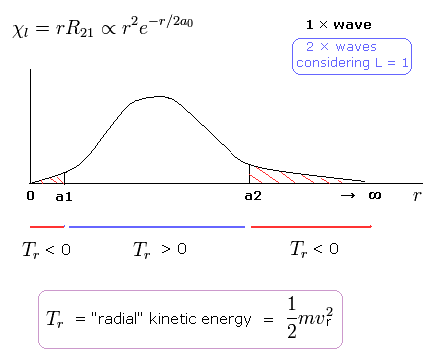As shown in Fig.21, the 2P "radial" wave function ( χ = rR21 ) contains the regions of the negative kinetic energy ( r < a1, a2 < r ).
When the r is bigger than a2, the Coulomb potential energy becomes higher than some maximum value.
In this region, to keep the total energy (E < 0) constant, the "radial" kinetic energy must be negative ( T < 0 ) !

Also in the region less than a1, radial kinetic energy becomes negative, though tangential kinetic energy (= angular momentum ) remains to be positive.